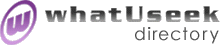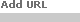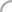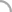SearchThe Directory The Webfor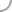ProbabilityNew! Submit a site

Categories:Journals (5)Mathematicians (21) Events (19)Research (5)

whatUseek Collection Sites (submit a site ):

Give your site great placement in this category in as little as two business days!

whatUseek Directory Site Listings:

A Compendium of Common Probability Distributions - A reference site describing many common probability distributions and their properties including, pdf, cdf, moments, random variate generation, etc.

An Introduction to Stochastics and Finance - Links to web sites related to financial mathematics : Introduction to stochastic analysis applied to finance, option pricing and risk management.

Applied Probability Trust - 4 titles.

Applied Probability and Stochastic Processes - An online course by Wlodzimierz Bryc in DVI, PS and HTML format.

ArXiv Front: PR Probability Theory - Probability section of the mathematics e-print arXiv

Bibliography for Computational Probability and Statistics - 300 references, journals home pages.

Calculus and Probability - Online course by Stefan Waner and Steven R. Costenoble

Highly Structured Stochastic Systems - An ESF network.

Imprecise Probabilities Project - To help advance the theory and applications of imprecise probabilities, mainly by the dissemination of relevant information.

Measure and Integration - Part of Dave Rusin's excellent Mathematical Atlas

Probabilistic Modeling Process - Decision making under risk is presented in the context of decision analysis using different decision criteria for public and private decisions based on decision criteria, type, and quality of available information together with risk assessment.

Probabilistic Thinking - By Richard Jeffrey. HTML.

Probability - Examines various definitions of Probability, and some paradoxes and perplexities.

Probability - Examines various definitions of Probability, and some paradoxes and perplexities.

Probability Abstract Service - Probability Abstract Letters are sent about once every two months. The subscription is free.

Probability Theory and Stochastic Processes - Part of Dave Rusin's excellent Mathematical Atlas.

Probability Theory: The Logic of Science - Online textbook by E.T. Jaynes in PS format.

Probability Tutorials - Tutorials in measure theory, lebesgue integration and probability, by Noel Vaillant

Probability and Random Process Class Notes - Class notes for a university course on probability and random processes, by John L. Stensby. Notes are in .pdf format. Introductory through advanced topics are covered.

Probability and Statistics - A personal page by Justin Smith dedicated to exploring interesting probability and statistical questions.

[ 1 2 ]Help build the largest human-edited directory on the web.About   Help   Content Filter   Terms   Privacy Policy© 2018 whatUseek